Function Repository Resource:

# GainRescale

Apply a flatter or steeper variation in the middle of an interval

Contributed by: Jan Mangaldan
 ResourceFunction["GainRescale"][a,x] biases x to be flatter towards the middle if a<1/2, and steeper towards the middle if a>1/2, as x runs from 0 to 1. ResourceFunction["GainRescale"][a,x,{min,max}] biases x as x runs from min to max. ResourceFunction["GainRescale"][a,x,{min,max},{ymin,ymax}] biases x as x runs from min to max, with the result rescaled to run from ymin to ymax.

## Details and Options

a can be any real number between 0 and 1.
ResourceFunction["GainRescale"] is a symmetrized version of the resource function BiasedRescale.
The result of ResourceFunction["GainRescale"][a,x,{min,max}] is always between 0 and 1.
ResourceFunction["GainRescale"] can take the Method option for the bias function to use. Possible settings for the Method option are "Schlick" (default) and "Perlin".
With the default option setting Method"Schlick", ResourceFunction["GainRescale"] uses Schlick's bias function x/((1/a-2)(1-x)+1).
With Method"Perlin", ResourceFunction["GainRescale"] uses Perlin's bias function x-log2(a).
ResourceFunction["GainRescale"] is commonly used in computer graphics.

## Examples

### Basic Examples (3)

Evaluate numerically:

 In:=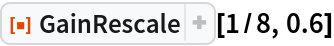Out=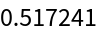Plot over a subset of the reals:

 In:=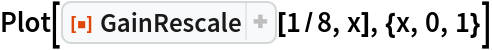Out=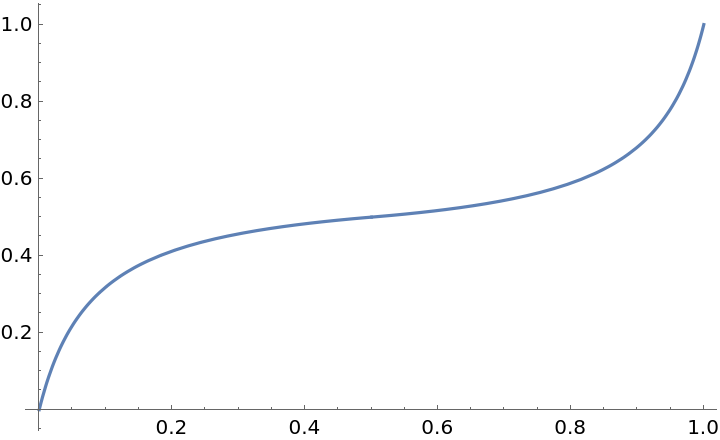Plot different gain functions:

 In:=Out=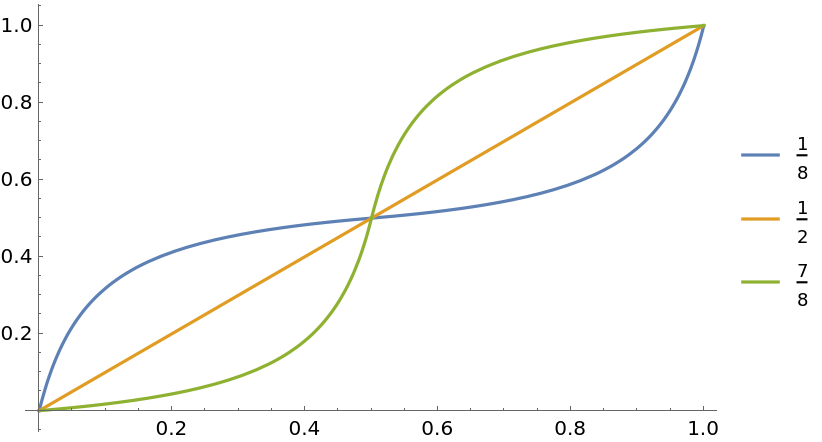### Scope (4)

Evaluate for symbolic x:

 In:=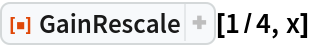Out=GainRescale threads over lists in its first and second arguments:

 In:=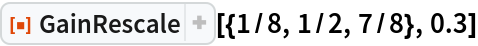Out=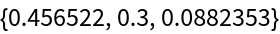In:=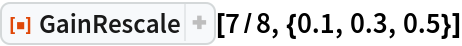Out=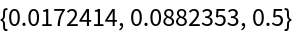Plot different gain functions with a rescaled domain:

 In:=Out=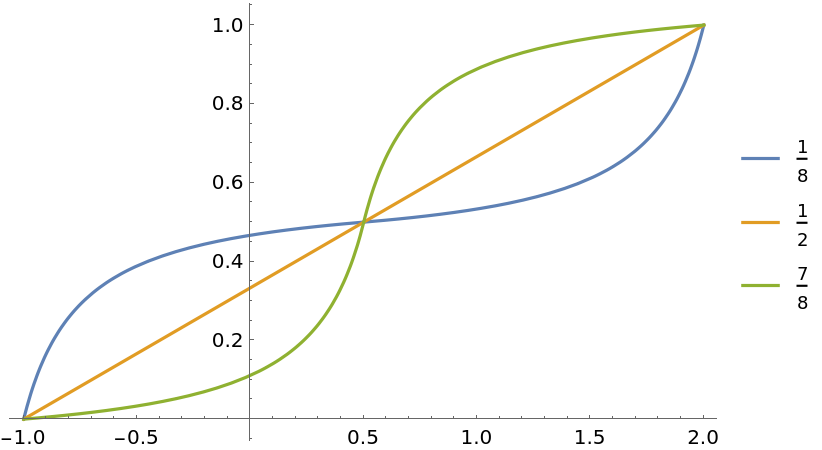Plot different gain functions with a rescaled domain and range:

 In:=Out=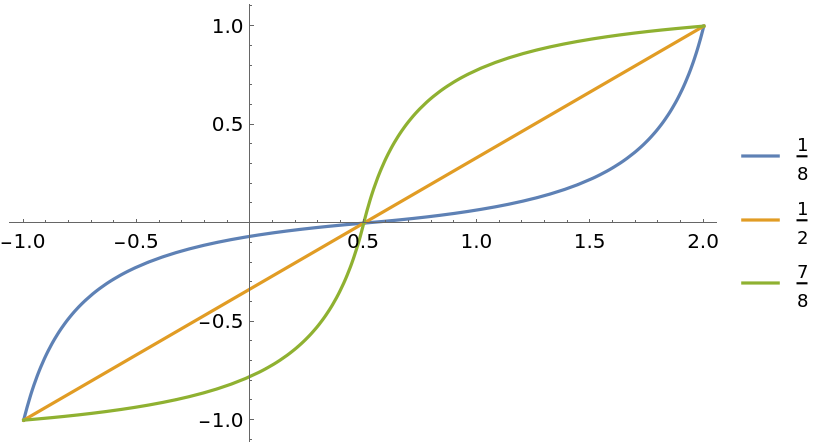### Options (1)

#### Method (1)

Compare the Schlick and Perlin gain functions:

 In:=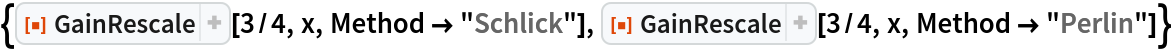Out=In:=Out=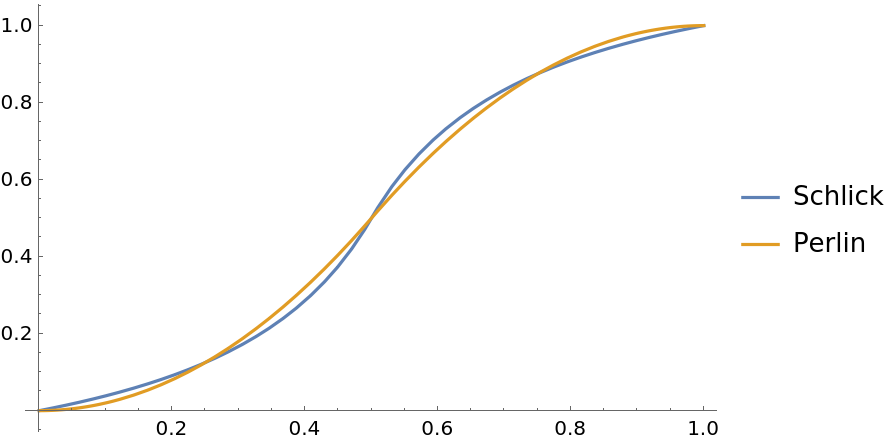### Applications (2)

 In:=Out=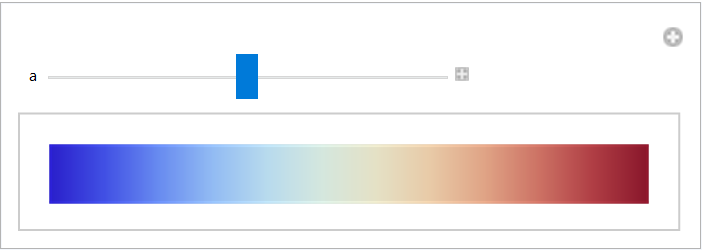Demonstrate the effect of GainRescale on easing in/easing out:

 In:=Out=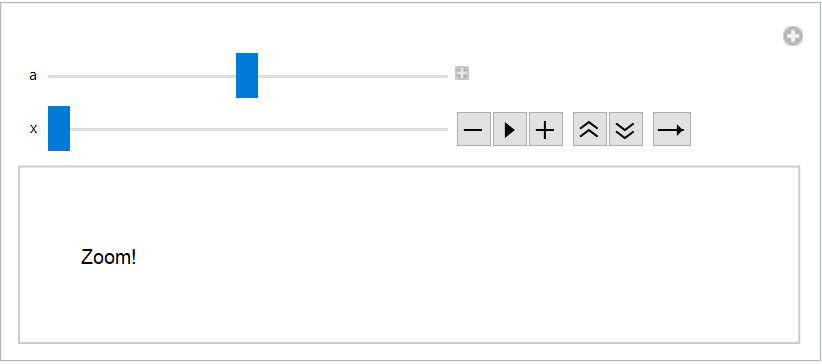### Properties and Relations (2)

GainRescale[1/2,x,{min,max}] is equivalent to Rescale[x,{min,max}]:

 In:=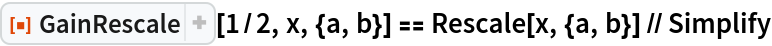Out=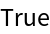GainRescale[a,x] is a symmetrized version of the resource function BiasedRescale, made symmetric about x=1/2:

 In:=Out=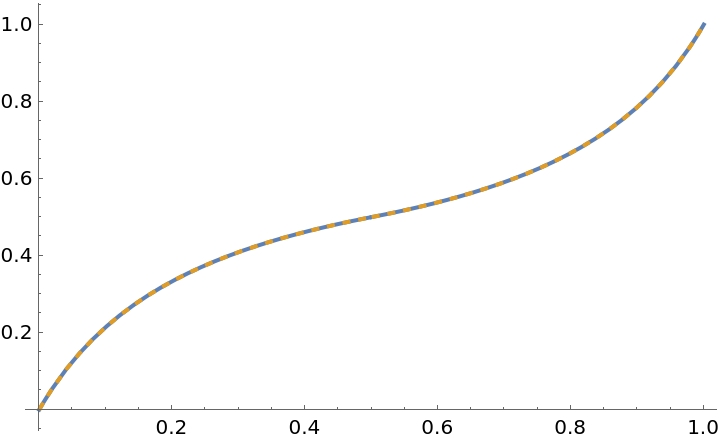### Possible Issues (2)

GainRescale is left unevaluated if the first argument is nonnumeric:

 In:=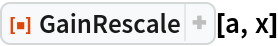Out=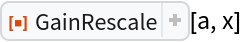GainRescale is left unevaluated if the first argument is not a real number between 0 and 1:

 In:=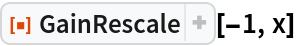Out=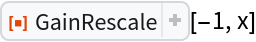## Version History

• 1.0.0 – 16 February 2021